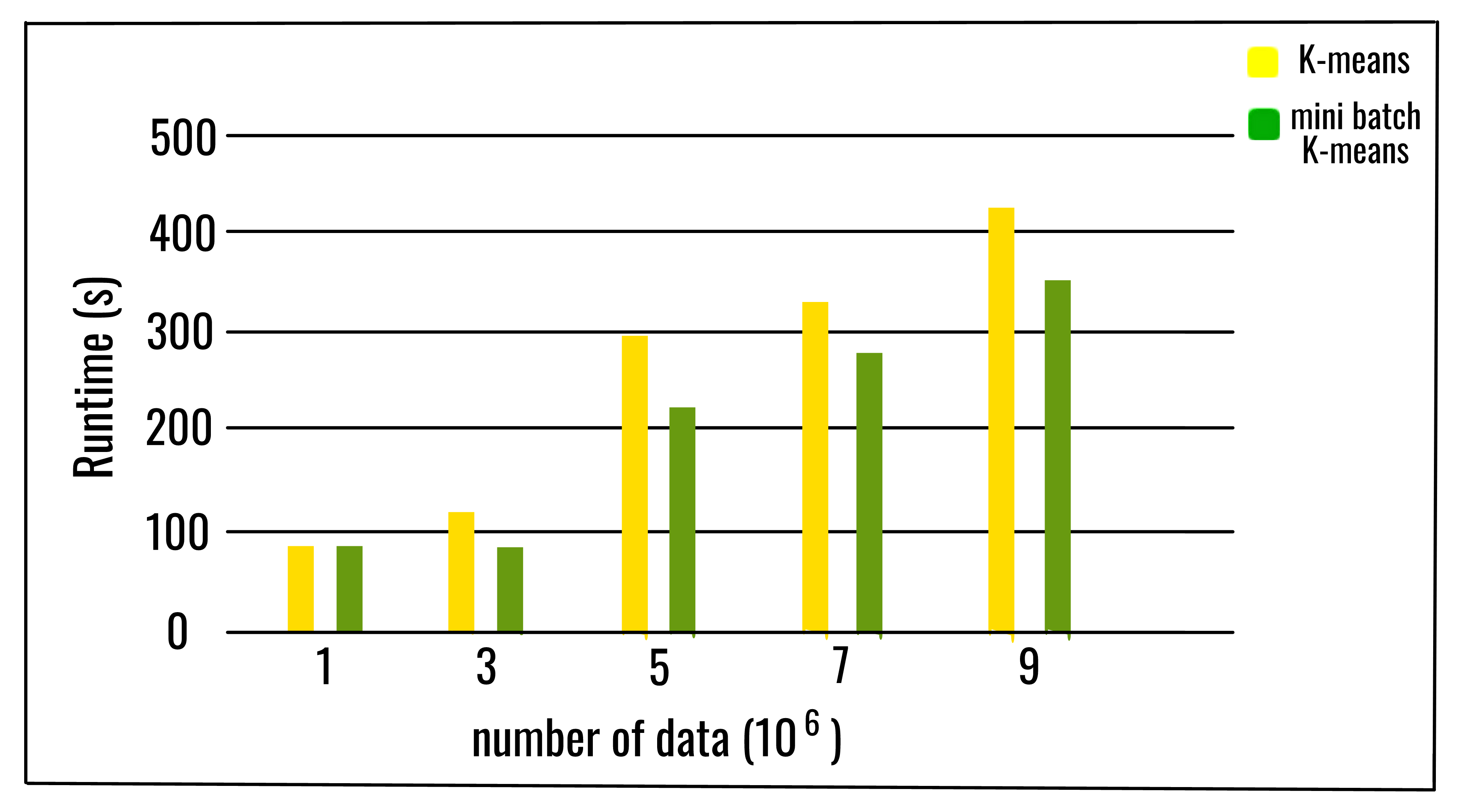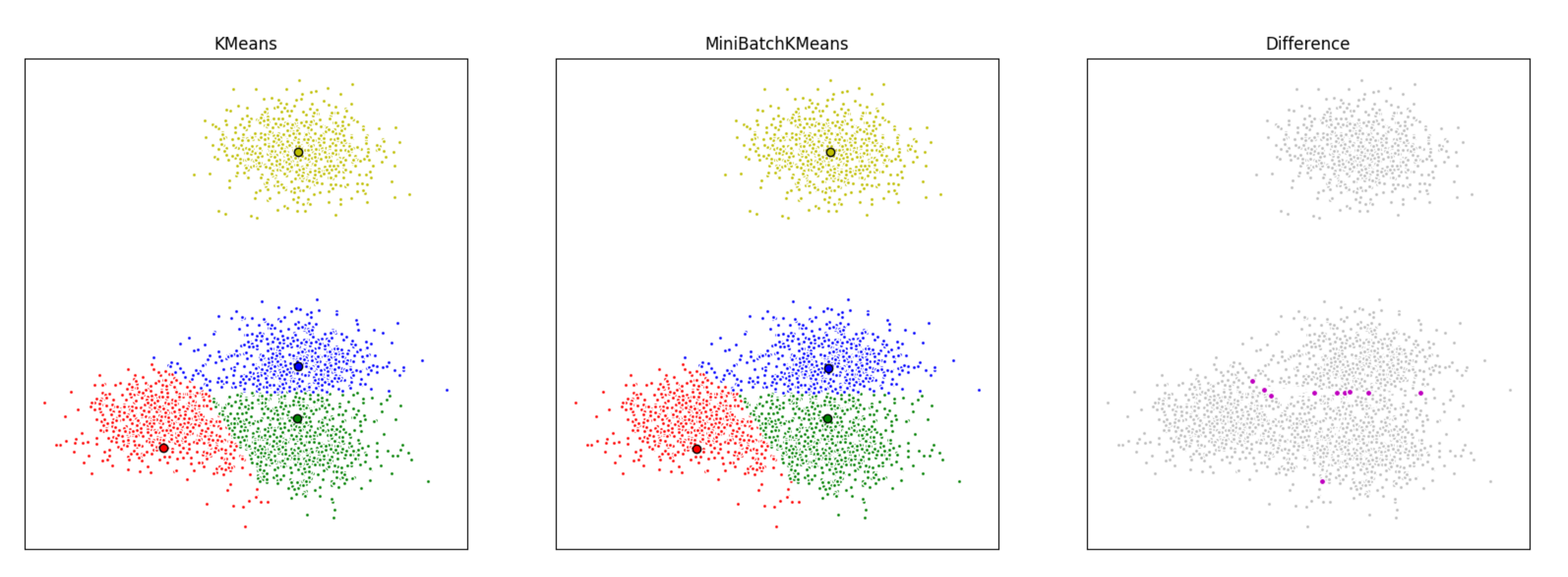# ML | Mini Batch K-means clustering algorithm

Prerequisite: Optimal value of K in K-Means Clustering

K-means is one of the most popular clustering algorithms, mainly because of its good time performance. With the increasing size of the datasets being analyzed, the computation time of K-means increases because of its constraint of needing the whole dataset in main memory. For this reason, several methods have been proposed to reduce the temporal and spatial cost of the algorithm. A different approach is the Mini batch K-means algorithm.

Mini Batch K-means algorithm‘s main idea is to use small random batches of data of a fixed size, so they can be stored in memory. Each iteration a new random sample from the dataset is obtained and used to update the clusters and this is repeated until convergence. Each mini batch updates the clusters using a convex combination of the values of the prototypes and the data, applying a learning rate that decreases with the number of iterations. This learning rate is the inverse of the number of data assigned to a cluster during the process. As the number of iterations increases, the effect of new data is reduced, so convergence can be detected when no changes in the clusters occur in several consecutive iterations.
The empirical results suggest that it can obtain a substantial saving of computational time at the expense of some loss of cluster quality, but not extensive study of the algorithm has been done to measure how the characteristics of the datasets, such as the number of clusters or its size, affect the partition quality.The algorithm takes small randomly chosen batches of the dataset for each iteration. Each data in the batch is assigned to the clusters, depending on the previous locations of the cluster centroids. It then updates the locations of cluster centroids based on the new points from the batch. The update is a gradient descent update, which is significantly faster than a normal Batch K-Means update.

Below is the algorithm for Mini batch K-means

```Given a dataset D = {d1, d2, d3, .....dn},
no. of iterations t,
batch size b,
no. of clusters k.

k clusters C = {c1, c2, c3, ......ck}

initialize k cluster centers O = {o1, o2, .......ok}
# _initialize each cluster
Ci = Φ (1=< i =< k)
# _initialize no. of data in each cluster
Nci = 0 (1=< i =< k)

for j=1 to t do:
# M is the batch dataset and xm
# is the sample randomly chosen from D
M = {xm | 1 =< m =< b}

# catch cluster center for each
# sample in the batch data set
for m=1 to b do:
oi(xm) = sum(xm)/|c|i (xm ε M and xm ε ci)
end for
# update the cluster center with each batch set

for m=1 to b do:
# get the cluster center for xm
oi = oi(xm)
# update number of data for each cluster center
Nci = Nci + 1
#calculate learning rate for each cluster center
lr=1/Nci
# take gradient step to update cluster center
oi = (1-lr)oi + lr*xm
end for
end for```

Python implementation of the above algorithm using scikit-learn library:

 `from` `sklearn.cluster ``import` `MiniBatchKMeans, KMeans ` `from` `sklearn.metrics.pairwise ``import` `pairwise_distances_argmin ` `from` `sklearn.datasets.samples_generator ``import` `make_blobs ` ` `  `# Load data in X  ` `batch_size ``=` `45` `centers ``=` `[[``1``, ``1``], [``-``2``, ``-``1``], [``1``, ``-``2``], [``1``, ``9``]] ` `n_clusters ``=` `len``(centers) ` `X, labels_true ``=` `make_blobs(n_samples ``=` `3000``, ` `                            ``centers ``=` `centers, ` `                            ``cluster_std ``=` `0.9``) ` ` `  `# perform the mini batch K-means ` `mbk ``=` `MiniBatchKMeans(init ``=``'k-means++'``, n_clusters ``=` `4``, ` `                      ``batch_size ``=` `batch_size, n_init ``=` `10``, ` `                      ``max_no_improvement ``=` `10``, verbose ``=` `0``) ` ` `  `mbk.fit(X) ` `mbk_means_cluster_centers ``=` `np.sort(mbk.cluster_centers_, axis ``=` `0``) ` `mbk_means_labels ``=` `pairwise_distances_argmin(X, mbk_means_cluster_centers) ` ` `  `# print the labels of each data ` `print``(mbk_means_labels) `

The mini batch K-means is faster but gives slightly different results than the normal batch K-means.
Here we cluster a set of data, first with K-means and then with mini batch K-means, and plot the results. We will also plot the points that are labeled differently between the two algorithms.As the number clusters and the number of data increases, the relative saving in computational time also increases. The saving in computational time is more noticeable only when the number of clusters is very large. The effect of the batch size in the computational time is also more evident when the number of clusters is larger. It can be concluded that, increasing the number of clusters, decreases the similarity of the mini batch K-means solution to the K-means solution. Despite that the agreement between the partitions decreases as the number of clusters increases, the objective function does not degrade at the same rate. It means that the final partitions are different, but closer in quality.

References:
https://upcommons.upc.edu/bitstream/handle/2117/23414/R13-8.pdf
https://scikit-learn.org/stable/modules/generated/sklearn.cluster.MiniBatchKMeans.html

My Personal Notes arrow_drop_upCheck out this Author's contributed articles.

If you like GeeksforGeeks and would like to contribute, you can also write an article using contribute.geeksforgeeks.org or mail your article to contribute@geeksforgeeks.org. See your article appearing on the GeeksforGeeks main page and help other Geeks.

Please Improve this article if you find anything incorrect by clicking on the "Improve Article" button below.

Article Tags :
Practice Tags :

2

Please write to us at contribute@geeksforgeeks.org to report any issue with the above content.